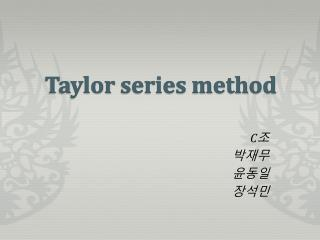DownloadDownload PresentationTaylor series method

# Taylor series method

Télécharger la présentation## Taylor series method

- - - - - - - - - - - - - - - - - - - - - - - - - - - E N D - - - - - - - - - - - - - - - - - - - - - - - - - - -
##### Presentation Transcript

1. Taylorseries method C조 박재무 윤동일 장석민

2. outline • What is Taylor seriesmethod • Main Topic 1 • Main Topic 2 • Future work Problem 1 Result 1 How to solve problem 1 Summary 1 Problem 2 Result 2 How to solve problem 2 Summary 2

3. WhatisTaylorseries? The Taylor series of a real or complex-valued functionƒ(x) that is infinitely differentiable at a real or complex number ais the power series which can be written in the more compact sigma notation as When a = 0, the series is also called a Maclaurin series

4. WhatisTaylorseries? Taylor seriesis a representation of a function as an infinite sum of terms that are calculated from the values of the function's derivative at a single point. Example

5. WhatisTaylorseries method? Euler’s method Tangent line local approximation Taylor series Higher degree better approximation

6. Graph of e^x Taylortool in matlab Taylor polynomial of degree 1 (Euler’s M) Taylor polynomial of degree 2

7. Problem 1 Compute the Taylor polynomials of degree 4 for the solutions to the given initial value problems. Use these Taylor polynomials to approximate the solution at x = 1.

8. Result 1 Problem(1) has error that can’t be ignored Problem(2) has large error

9. Subproblem1(Numerical)

10. Subproblem 1 (Analytical) Integrating factor

11. Subproblem 2 (numerical)

12. Subproblem 2(Analytic)

13. How can we decrease the error? Problem (1) : Increase the degree of Taylor polynomial Error : 0.247498 Error : 0.000298

14. How can we decrease the error? Problem (2) Can not be solve the problem by increasing degree…..

15. How can we decrease the error? Problem (2) Euler’s method can decrease the error When mesh size = 0.1, y(1) = 2.094317 errer = 0.050841 x=0; y=4; for i=1:100 yp=y*(2-y); y1=y+0.1*yp; y=y1; x=x+0.01; end

16. Solve D.E in matlab syms x y f=dsolve('Dy=x-2*y', 'y(0)=1', 'x') p=taylor(f,5,x) f = x/2 + 5/(4*exp(2*x)) - ¼ p = (5*x^4)/6 - (5*x^3)/3 + (5*x^2)/2 - 2*x + 1

17. Summary 1 Taylor series method can find approximation But it need higher degree and some points in some function can not enable to get approximation

18. Problem 2 Compare the use of Euler’s method with that of Taylor series to approximate the solution (x) to the initial value problem Do this by completing the following table:

19. Result 2(X)

20. Result 2

21. Result 2(Error)

22. Problem 2 ( Euler’s method) x=0; y=0; for i=1:10  for i=1:100 slope=-y+cos(x)-sin(x); y1=y+0.1*slope;  y1=y+0.3*slope; y=y1; x=x+0.1;  x=x+0.3; end

23. Problem 2 ( Taylor’s method)(X)

24. Problem 2 ( Taylor’s method)(x) Step size = 1 Step size = 3 Step size = 1 Step size = 3

25. Problem 2 ( Taylor’s method)

26. Problem 2 ( Taylor’s method) Taylor polynomial Degree 2 Taylor polynomial Degree 5

27. Graph(degree 2) y=cos(x)-exp(-x) y=cos(x)-exp(-x) Taylor polynomial of degree 2 with step size 0.1 Taylor polynomial of degree 2 with step size 1

28. Problem 2 ( Taylor’s method) Degree: 2 Degree: 5 x=0; y=0; step=0.1; for i=1:10 slope=-y+cos(x)-sin(x); slope2=-2*cos(x)+y; slope3=cos(x)+sin(x)-y; slope4=y; slope5=-y+cos(x)-sin(x); y1=y+step*slope +(step)^2/2*slope2 +(step)^3/(3*2*1)*slope3 +(step)^4/(4*3*2*2)*slope4 +(step)^5/(5*4*3*2*1)*slope5; y=y1; x=x+step; end x=0; y=0; step=0.1; for i=1:10 slope=-y+cos(x)-sin(x); slope2=-2*cos(x)+y; y1=y+step*slope+ (step)^2/2*slope2; y=y1; x=x+step; end

29. Convergence rate

30. Problem 2 ( Analytical) Integrating factor

31. Problem 2 ( Analytical)

32. Summary 2 Good approximation In same method - Step size : small In same step size - Taylor Method is better than Euler’s Method - Taylor polynomial of higher degree is better than Taylor polynomial of lower degree But Taylor polynomial of higher degree needs many calculation

33. Think more…. How can we get approximation fast and accurate with simple code(calculation)? Runge-Kutta